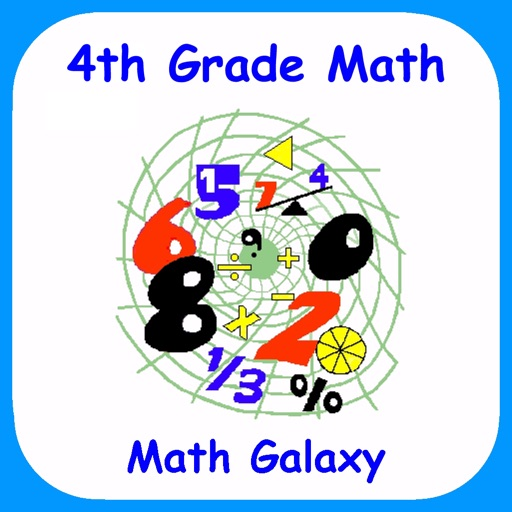## "I love all of the Math Galaxy apps for their ease of use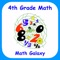# 4th Grade Math - Math Galaxy

by Math Galaxy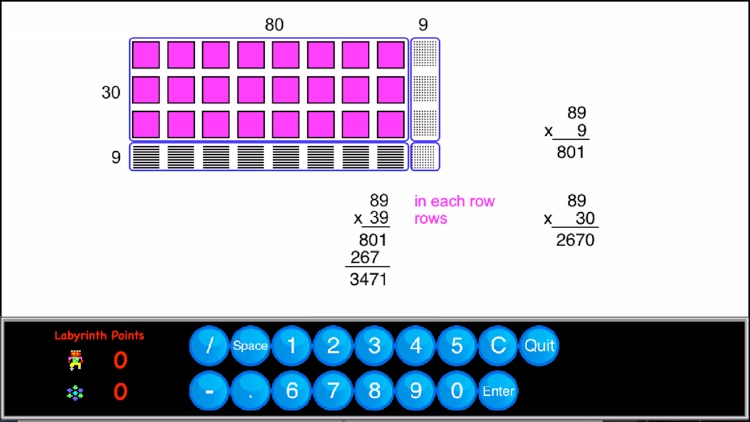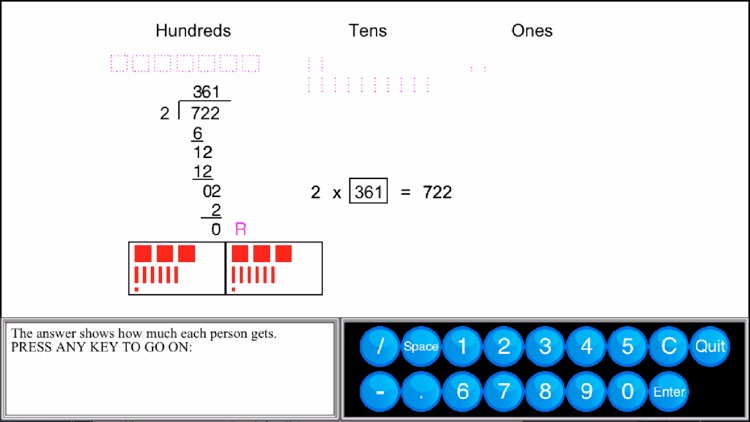"I love all of the Math Galaxy apps for their ease of use."### App Details

Version
4.0
Rating
(3)
Size
341Mb
Genre
Education Casual Board
Last updated
March 23, 2021
Release date
September 13, 2014

### App Screenshots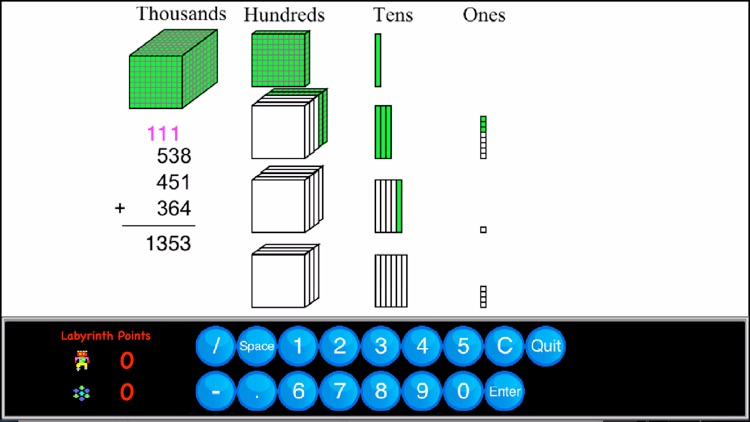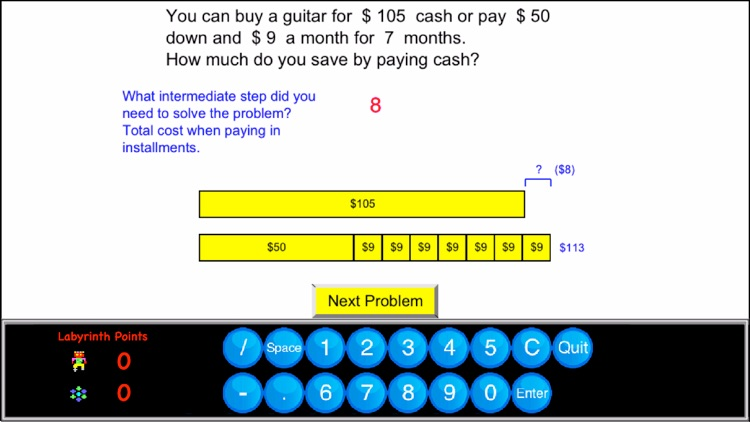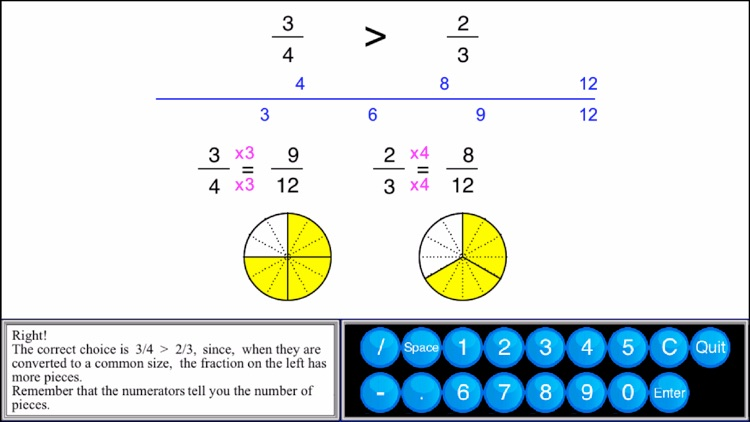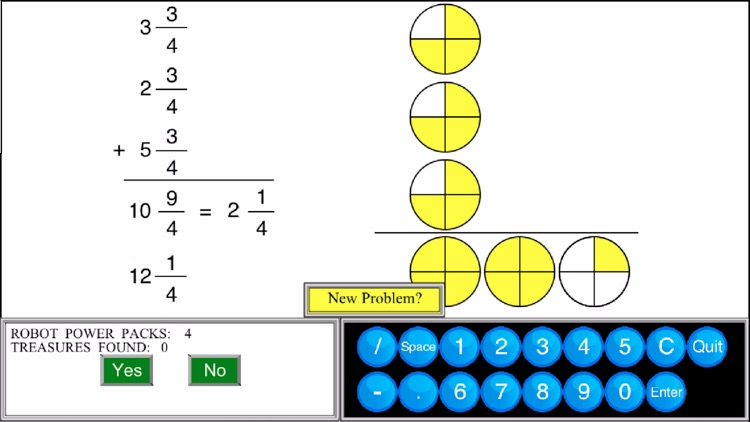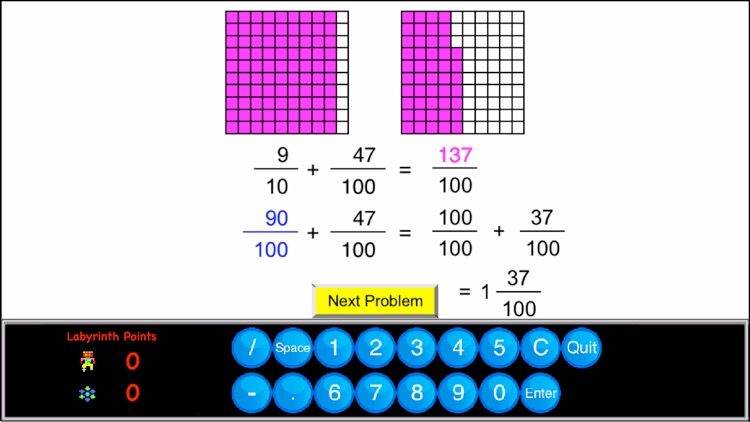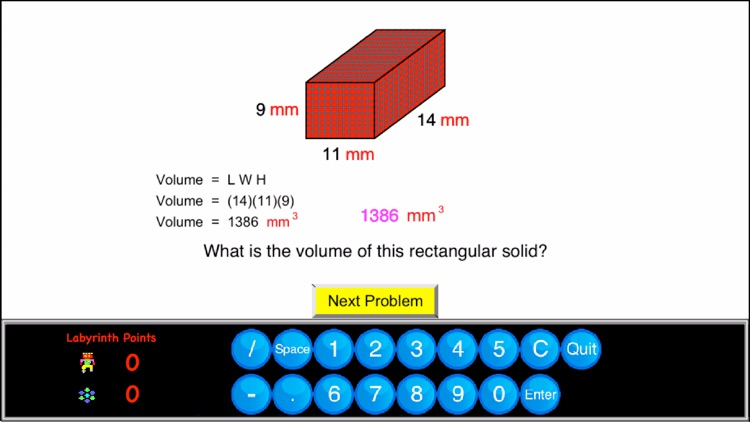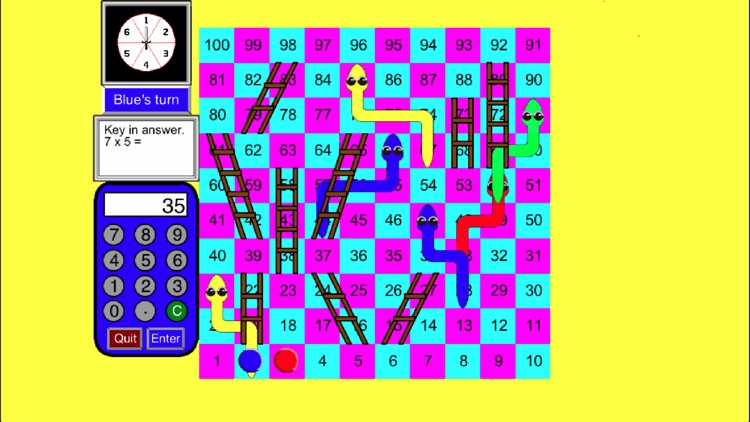### App Store Description

"I love all of the Math Galaxy apps for their ease of use."

See the rest of the review at:

In fourth grade math the emphasis is on multi-digit multiplication and division with multi-digit dividends, developing an understanding of fractions and decimal fractions, plus developing fluency in addition and subtraction.

Math Galaxy’s Fourth Grade Math covers all of the Common Core math standards for fourth grade as listed below.
The concepts are presented in bite-size pieces with an unlimited supply of problems, with numbers chosen randomly.
Illustrations are used throughout to reinforce the concepts.
You can be guided through problems step-by-step or just give final answers.
By solving problems students earn robots to use in a maze game, plus there are several other games involving math practice.
Certificates with scores and summaries can be emailed from final-answer mode to be printed.
A stylus is recommended for iphones.

Topics covered are listed below along with their Common Core standard.
More details on common core standards can be found at: http://www.corestandards.org/Math/

NUMBERS AND OPERATIONS IN BASE 10 (4.NBT)

Generalize place value understanding for multi-digit whole numbers.
Use place value to perform multi-digit arithmetic.

Place Value Blocks (4.NBT.1)

Place Value (4.NBT.2)
Place Names
Expanded Notation
Comparing Numbers
Ordering Numbers

Rounding & Estimating (4.NBT.3)

Alternative Method - Partial Sums
Alternative Method - Expanded Notation

Subtracting Whole Numbers (4.NBT.4)
3-4-Digit Subtraction

Multiplying Whole Numbers (4.NBT.5)
1-Digit x 2, 3, or 4-Digits
2-Digit x 2-Digit
Mult & Div by 10's

Dividing Whole Numbers (4.MBT.6)
Relating Division to Multiplication
1-Digit Divisor, 2-4-Digit Dividend
Zero’s in Quotient
Missing Number

OPERATIONS AND ALGEBRAIC THINKING (4.OA)

Use the four operations with whole numbers to solve problems.
Gain familiarity with factors and multiples.

Multiplicative Comparison (4.OA.1, 2)
Word Problems (4.OA.2)
Multi-Step Word Problems (4.OA.3)
Factors & Prime Numbers (4.OA.4)
Patterns (4.OA.5)

NUMBER AND OPERATIONS - FRACTIONS (4.NF)

Extend understanding of fraction equivalence and ordering.
Understand decimal notation for fractions and compare decimal fractions.

Identifying Fractions (4.NF.1)
Fractions on # Line (4.NF.1)
Part of a Group (4.NF.1)
Equivalent Fractions (4.NF.1)
Comparing Fractions (4.NF.2)
Reducing & Improper Fractions (4.NF..3a)
Adding & Subtracting Like Fractions (4.NF.3b)
Multiplying Fractions (4.NF.4)
Fractions Word Problems (4.NF.3c, 4.NF.4c)

Writing Decimals (4.NF.6)
Decimals on # Line (4.NF.6)
Comparing Decimals (4.NF.7)
Ordering Decimals (4.NF.7)

MEASUREMENT AND DATA (4.MD)

Solve problems involving measurement and conversion of measurements.

Measurement Conversions (4.MD.1,2)
Length (4.MD.1,2)
Area & Perimeter (4.MD.1-3)
Volume (4.MD.1,2)
Liquid Volume (4.MD.1,2)
Line Plots (4.MD.4)
Mass (in Riddles game) (4.MD.1,2)
Time (in Riddles game) (4.MD.1,2)
Angles (4.MD.5-7) under Geometry

GEOMETRY (4.G)

Draw and identify lines and angles, and classify shapes.

Terminology (4.G.1-3)
Measuring Angles
Angles (4.G.1-3)

Games

The Money Trail
Bridge the Swamp
Labyrinth (a maze game - robots are earned in exercises.)